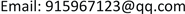1北京建筑大学测绘与城市空间信息学院，北京

2北京未来城市设计高精尖创新中心，北京

3武汉大学测绘学院，湖北 武汉1. 引言

2. 原理与方法2.1. 最小二乘法

( x − a ) 2 + ( y − b ) 2 + ( z − c ) 2 = r 2 (1)

x 2 + y 2 + z 2 − 2 a x − 2 b y − 2 c z + a 2 + b 2 + c 2 = r 2 (2)

x 2 + y 2 + z 2 − A x − B y − C z + D = 0 (3)

a = A 2 ,     b = B 2 ,     c = C 2 ,     r = a 2 + b 2 + c 2 − D (4)

2.2. 随机采样算法

Random sampling algorithm parameter

P离群值自由子集的期望概率
S估计模型M所用数据元素的最小数量
τ阈值

N维球体被定义为满足以下公式的一组点：

( p − c ) T ( p − c ) = r 2 (5)

∀ i , j       ( p i − c ) T ( p i − c ) = ( p j − c ) T ( p j − c ) = r 2 (6)

( p i − p j ) T c = 0.5 ( p i T p i − p j T p j ) (7)

[ ( p 1 x − p 2 x ) ( p 1 y − p 2 y ) ( p 1 x − p 3 x ) ( p 1 y − p 3 y ) ] [ c x c y ] = 0.5 [ ( p 1 x 2 − p 2 x 2 ) + ( p 1 x 2 − p 2 y 2 ) ( p 1 x 2 − p 3 x 2 ) + ( p 1 x 2 − p 3 y 2 ) ] (8)

A = ( a b c d ) , A − 1 = 1 a d − b c [ d − b − c a ] (9)

A = [ a b c d e f g h i ] ,     A − 1 = 1 a ( e i − f h ) − b ( d i − g h ) + c ( d h − e g ) [ e i − f h c h − b i b f − c e f g − d i a i − c g c d − a f d h − e g b g − a h a e − a h ] (11)

δ i = p i T p i − 2 p i T c + c T c − r 2 (12)

[ c * , r * ] = arg min c , r ∑ i = 1 m δ i 2 (13)

∑ i = 1 m δ i 2 = ‖ A x − b ‖ 2 (15)

3. 实验与分析

“全站仪 + 最小二乘法”是利用全站仪采集螺栓球体表面上的4个随机点，然后通过MATLAB编写的最小二乘法程序对数据进行计算，最终得到螺栓球的半径和球体中心坐标。

RANSAC processing results (unit: m

Least squares processing results (unit: m

4. 实际应用

5. 结论